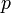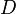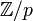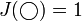# 06-1350/Homework Assignment 1

Solve the following problems and submit them in class by October 19, 2006:

1. Let$p$ be an odd prime. A knot diagram$D$ is called$p$-colourable if there is a non-constant map ("colouring") from the arcs of$D$ to${\mathbb Z}/p$ so that at every crossing, the average of the colours of the two "under" arcs is equal to the colour of the "over" arc (calculations in${\mathbb Z}/p$, of course).
1. Show that the newly-defined meaning of 3-colourable coincides with the definition given for this notion in class.
2. Show that "being$p$-colourable" is invariant under Reidemeister moves and hence defines a knot invariant.
3. (Hard and not mandatory) Prove that the (5,3) torus knot T(5,3) (pictured above) is not$p$-colourable for any$p$.
2. Use the recursion formula Failed to parse (unknown function\overcrossing): q^{-1}J(\overcrossing)-qJ(\undercrossing)=(q^{1/2} - q^{-1/2})J(\smoothing)
and the initial condition$J(\bigcirc)=1$ to compute the Jones polynomial Failed to parse (unknown function\HopfLink): J(\HopfLink)
of the Hopf link and the Jones polynomial Failed to parse (unknown function\righttrefoil): J(\righttrefoil)
of the right handed trefoil knot.

1. Explain in detail why is the set {knots of genus 3} definable using knotted trivalent graphs.
2. Explain in detail why is the set {knots of unknotting number 3} definable using knotted trivalent graphs.

Mandatory but unenforced. Find yourself in the class photo and identify yourself as explained in the photo page.